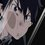# HOW TO expand determinants

• To expand $2\times 2$ Determinant

$X=\begin{vmatrix} a & b \\ c & d \end{vmatrix}\\ X=ad-bc$

practice here

• To expand $3\times 3$ Determinant

you can use the first row to expand the determinant by multiplying each element in the first raw by its sign [${ (-1) }^{ (R+C) }$ when R is the row number and C is the column number] by the remaining $2\times 2$determinant [delete the elements which have the same row number and the same column number and put the remaining element as $2\times 2$ determinant] then add the three results.

$X=\left| \begin{matrix} a & b & c \\ d & e & f \\ g & h & i \end{matrix} \right| \\ X=\left| \begin{matrix} { a }_{ 11 } & { b }_{ 12 } & { c }_{ 13 } \\ { d }_{ 21 } & { e }_{ 22 } & { f }_{ 23 } \\ { g }_{ 31 } & { h }_{ 32 } & { i }_{ 33 } \end{matrix} \right| \\ X={ (-1) }^{ (1+1) }a\times \begin{vmatrix} e & f \\ h & i \end{vmatrix}+{ (-1) }^{ (1+2) }b\begin{vmatrix} d & f \\ g & i \end{vmatrix}+{ (-1) }^{ (1+3) }c\begin{vmatrix} d & e \\ g & h \end{vmatrix}\\ X=a\times \begin{vmatrix} e & f \\ h & i \end{vmatrix}-b\begin{vmatrix} d & f \\ g & i \end{vmatrix}+c\begin{vmatrix} d & e \\ g & h \end{vmatrix}\\ X=a\times (ei-fh)-b\times (di-fg)+c\times (dh-eg)$

NOTE: When you expand, you can use any row or any column for example you can expand the above determinant by the second column as:

$X=\left| \begin{matrix} a & b & c \\ d & e & f \\ g & h & i \end{matrix} \right| \\ X=\left| \begin{matrix} { a }_{ 11 } & { b }_{ 12 } & { c }_{ 13 } \\ { d }_{ 21 } & { e }_{ 22 } & { f }_{ 23 } \\ { g }_{ 31 } & { h }_{ 32 } & { i }_{ 33 } \end{matrix} \right| \\ X={ (-1) }^{ (1+2) }b\times \begin{vmatrix} d & f \\ g & i \end{vmatrix}+{ (-1) }^{ (2+2) }e\begin{vmatrix} a & c \\ g & i \end{vmatrix}+{ (-1) }^{ (3+2) }h\begin{vmatrix} a & c \\ d & f \end{vmatrix}\\ X=-b\times \begin{vmatrix} d & f \\ g & i \end{vmatrix}+e\begin{vmatrix} a & c \\ g & i \end{vmatrix}-h\begin{vmatrix} a & c \\ d & f \end{vmatrix}\\ X=-b\times (di-fg)+e\times (ai-cg)-h\times (af-cd)$

practice here

• To solve more than $3\times 3$ determinants

An easy way to solve more than $3\times 3$ determinant is converting it to triangular or diagonal determinant

1- Triangular determinant:

a. Upper triangular determinant: Elements which are under the main diagonal are zero $X=\begin{vmatrix} a & b & c & d \\ 0 & f & g & h \\ 0 & 0 & k & l \\ 0 & 0 & 0 & p \end{vmatrix}\\ X=a\times f\times k\times p$

b. Lower triangular determinant: Elements which are above the main diagonal are zero $X=\begin{vmatrix} a & 0 & 0 & 0 \\ e & f & 0 & 0 \\ i & j & k & 0 \\ m & n & o & p \end{vmatrix}\\ \\ X=a\times f\times k\times p$

2- Diagonal determinant:Elements which are under and above the main diagonal are zero $X=\begin{vmatrix} a & 0 & 0 & 0 \\ 0 & f & 0 & 0 \\ 0 & 0 & k & 0 \\ 0 & 0 & 0 & p \end{vmatrix}\\ \\ X=a\times f\times k\times p$

practice hereNote by Abdulrahman El Shafei
6 years, 7 months ago

This discussion board is a place to discuss our Daily Challenges and the math and science related to those challenges. Explanations are more than just a solution — they should explain the steps and thinking strategies that you used to obtain the solution. Comments should further the discussion of math and science.

When posting on Brilliant:

• Use the emojis to react to an explanation, whether you're congratulating a job well done , or just really confused .
• Ask specific questions about the challenge or the steps in somebody's explanation. Well-posed questions can add a lot to the discussion, but posting "I don't understand!" doesn't help anyone.
• Try to contribute something new to the discussion, whether it is an extension, generalization or other idea related to the challenge.

MarkdownAppears as
*italics* or _italics_ italics
**bold** or __bold__ bold
- bulleted- list
• bulleted
• list
1. numbered2. list
1. numbered
2. list
Note: you must add a full line of space before and after lists for them to show up correctly
paragraph 1paragraph 2

paragraph 1

paragraph 2

[example link](https://brilliant.org)example link
> This is a quote
This is a quote
    # I indented these lines
# 4 spaces, and now they show
# up as a code block.

print "hello world"
# I indented these lines
# 4 spaces, and now they show
# up as a code block.

print "hello world"
MathAppears as
Remember to wrap math in $$ ... $$ or $ ... $ to ensure proper formatting.
2 \times 3 $2 \times 3$
2^{34} $2^{34}$
a_{i-1} $a_{i-1}$
\frac{2}{3} $\frac{2}{3}$
\sqrt{2} $\sqrt{2}$
\sum_{i=1}^3 $\sum_{i=1}^3$
\sin \theta $\sin \theta$
\boxed{123} $\boxed{123}$

Sort by:

Brilliant WIKI's are waiting for this contribution

- 6 years, 7 months ago

How can I contribute by this note ?

- 6 years, 6 months ago

@Calvin Lin How would you do that?

- 6 years, 6 months ago

nice note @abdulrahman khaled

- 6 years, 6 months ago

Thanks :-)

- 6 years, 6 months ago